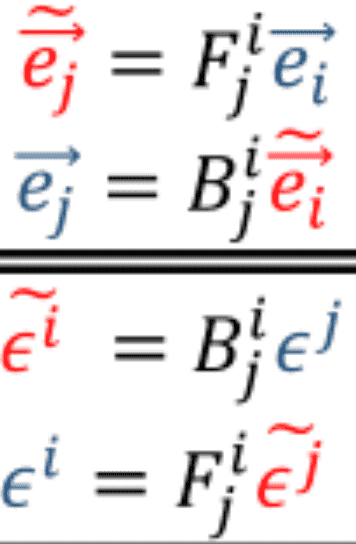# Transformation Rules For A General Tensor M

• B
• Vanilla Gorilla

#### Vanilla Gorilla

TL;DR Summary
Is it correct to say that a bunch of tensor products of all the basis vectors and covectors composing a general tensor M, multiplied by the components of M, lead to the transformation rule for M?
So, I've been watching eigenchris's video series "Tensors for Beginners" on YouTube. I am currently on video 14. I am a complete beginner and just want some clarification on if I'm truly understanding the material.
Basically, is everything below this correct?

In summary of the derivation of the transformation rules for a general tensor $$M$$, using algebraic notation, we just write the product of the components of the tensor $$M_{c_1, ~ c_2, ~ c_3,... ~ c_g}^{v_1, ~ v_2, ~ v_3,... ~ v_h}$$, with its basis vectors and basis covectors, where $$\vec {e}_{subscript}$$ represents basis vectors, and $$\epsilon^{superscript}$$ represents basis covectors.
This looks like $$\large {M = M_{c_1, ~ c_2, ~ c_3,... ~ c_g}^{v_1, ~ v_2, ~ v_3,... ~ v_h} \otimes_{n=1}^h {\vec {e}_{n_1}} \otimes_{p=1}^g {\epsilon^{p_1}}}$$
Where $$\large {\otimes_{n=1}^h {\vec {e}_{n_1}} \otimes_{p=1}^g {\epsilon^{p_1}}}$$ is basically just representative of a bunch of tensor products of all the basis vectors and covectors which compose $$M$$ in reference to the definition of tensors as(Note that I use the notation $$\otimes_{n=1}^h$$ to denote a series of tensor products from $$n=1$$ to $$h$$)
Then, we use the covector and vector rules given here,Substitute those into the formula given previously,
$$\large {M = M_{c_1, ~ c_2, ~ c_3,... ~ c_g}^{v_1, ~ v_2, ~ v_3,... ~ v_h} \otimes_{n=1}^h {\vec {e}_{n_1}} \otimes_{p=1}^g {\epsilon^{p_1}}}$$
Use linearity to bring the forward and/or backward transforms next to $$M_{c_1, ~ c_2, ~ c_3,... ~ c_g}^{v_1, ~ v_2, ~ v_3,... ~ v_h}$$, with the backward transforms in front of $$M_{c_1, ~ c_2, ~ c_3,... ~ c_g}^{v_1, ~ v_2, ~ v_3,... ~ v_h}$$, and the forward transforms behind it. I note this detail because I'm 99% sure we don't have commutativity with tensors in general; please correct me if I'm wrong about that, though!
All these transforms in conjunction act on $$M_{c_1, ~ c_2, ~ c_3,... ~ c_g}^{v_1, ~ v_2, ~ v_3,... ~ v_h}$$ to give $$\tilde {M}_{c_1, ~ c_2, ~ c_3,... ~ c_g}^{v_1, ~ v_2, ~ v_3,... ~ v_h}$$
The same general process can be done in reverse to convert $$\tilde {M}_{c_1, ~ c_2, ~ c_3,... ~ c_g}^{v_1, ~ v_2, ~ v_3,... ~ v_h}$$ to give $$M_{c_1, ~ c_2, ~ c_3,... ~ c_g}^{v_1, ~ v_2, ~ v_3,... ~ v_h}$$

P.S., I'm not always great at articulating my thoughts, so my apologies if this question isn't clear.